### IMO Shortlist 1994 problem C4

Kvaliteta:
Avg: 0,0
Težina:
Avg: 7,0
There are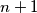$n + 1$ cells in a row labeled from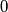$0$ to$n$ and$n + 1$ cards labeled from$0$ to$n$. The cards are arbitrarily placed in the cells, one per cell. The objective is to get card$i$ into cell$i$ for each$i$. The allowed move is to find the smallest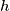$h$ such that cell$h$ has a card with a label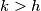$k > h$, pick up that card, slide the cards in cells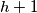$h + 1$,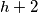$h + 2$, ... ,$k$ one cell to the left and to place card$k$ in cell$k$. Show that at most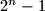$2^n - 1$ moves are required to get every card into the correct cell and that there is a unique starting position which requires$2^n - 1$ moves. [For example, if \$n = 2\$ and the initial position is 210, then we get 102, then 012, a total of 2 moves.]
Izvor: Međunarodna matematička olimpijada, shortlist 1994